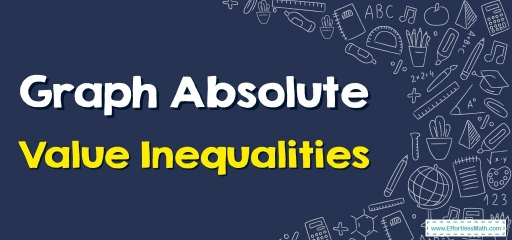# How to Graph Absolute Value Inequalities?

To graph an absolute value inequality, first, you need to solve the absolute value inequality.The absolute value of a number describes its distance from zero. To graph absolute value inequality you must first solve the inequality and then represent absolute value inequalities on a number line.

## A step-by-step guide to graph absolute value inequalities

• Isolate the absolute value expression.
• Write the equivalent compound inequality.
• Find two values.
• Draw a number line and Place dots or open dots on the two points corresponding to the solutions. if the symbol is ($$≥$$ or $$≤$$) then you fill in the dot if the symbol is ($$>$$ or $$<$$) then you do not fill in the dot.
• Sketch a line and show all numbers the variable can be.

### Graphing Absolute Value Inequalities– Example 1:

Solve and graph $$|3x-3|<9$$.

Solution:

Use absolute properties: if $$|a|<b$$, $$b > 0$$ then: $$-b<a<b → -9 < 3x-3 < 9$$

Add $$3$$ to each side of inequality: $$-9+3 < 3x-3+3 < 9+3 → -6 < 3x < 12$$

Divide each side of inequality by $$3$$: $$-2 < x < 4$$

Graph it on a number line.

### Graphing Absolute Value Inequalities– Example 2:

Solve and graph $$|x+2| ≥ 3$$.

Solution:

Split into two inequalities: $$x+2 ≥ 3$$ or $$x+2 ≤ -3$$.

Subtract $$2$$ from each side of each inequality:

$$x+2-2 ≥ 3-2$$ → $$x ≥ 1$$

or

$$x+2-2 ≤ -3-2$$ → $$x ≤ -5$$

$$x ≥ 1$$ or $$x ≤ -5$$.

Graph the numbers that satisfy both conditions:

## Exercises for Graph Absolute Value Inequalities

### Solve and graph the following inequalities.

• $$\color{blue}{4|x| ≥ 12}$$
• $$\color{blue}{|x-2| < 1}$$
• $$\color{blue}{|x+2| ≥ 2}$$
• $$\color{blue}{5|x+2| ≥ 15}$$

• $$\color{blue}{4|x| ≥ 12}$$
• $$\color{blue}{|x-2| < 1}$$
• $$\color{blue}{|x+2| ≥ 2}$$
• $$\color{blue}{5|x+2| ≥ 15}$$

### What people say about "How to Graph Absolute Value Inequalities? - Effortless Math: We Help Students Learn to LOVE Mathematics"?

No one replied yet.

X
30% OFF

Limited time only!

Save Over 30%

SAVE $5 It was$16.99 now it is \$11.99